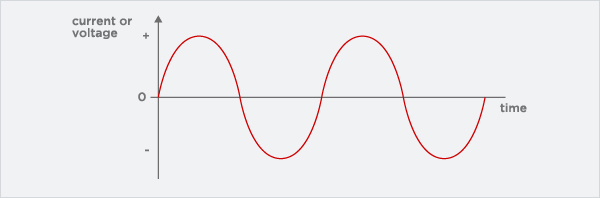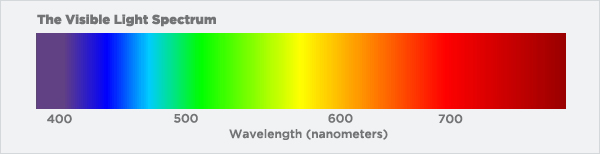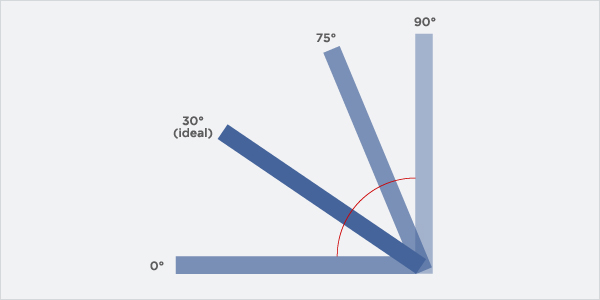## Glossary of Terms

Alternating current (AC) - A type of electrical current which works by reversing the direction of the electricity in regular cycles. This is used most commonly because the voltage can be controlled relatively easily.Amorphous silicon - A chemical element with no crystaline structure, manufactured by depositing layers of doped silicon on substrate.

Ampere (amp) - A unit used to measure electrical current, measured by the number of electrons passing one given point at a given time.

Angle of incidence - The angle at which a ray of sun hits the solar panel measured from perpendicular to it's surface. Electricity generation is at its maximum when the angle of incidence is zero, when the solar panel directly faces the sun.Annual solar savings - The amount of energy saved from having a solar panel feature. Calculated by comparing energy use with an identical building with no solar panel feature.

Anti-reflection coating - A thin coating applied to a solarcell surface to reduce light reflection, which will increase the amount of light transmission.

BIPV (Building-integrated photovoltaics) - The integration of photovoltaic (PV) technology into a building's structure. Eg. Replacing skylight systems with PV technologyChemical vapour deposition - When certain types of PV cells are made from depositing thin semiconductor films. In this, vapourised compounds which have desirable components are forced onto a substrate, causing the desired reaction to occur on the substrate.

Crystalline silicon - A type of photovoltaic cell made from a slice of single-crystal silicon or polycrystalline silicon.

Current at maximum power (IMP) - The level of electrical current at which maximum power is available from a module.

Cutoff voltage - The levels of voltage at which the controller takes the PV array from the battery or switches the battery off.

Direct current (DC) - A type of electrical current which travels in one direction, usually relatively low voltage and high current. Must be converted to AC for most household appliances.Dopant - An impurity added in small amounts to a pure semiconductor to change it and either introduce more electrons (n-dopant) or cause holes (p-dopant).

Electrical circuit - A closed path followed by electrons from a power source, through an electrical system and then back to the source, in a continuous circuit.

Electrical current - The flow of electrical energy (electricity), typically measured in amperes.

Electrical grid - An integrated system of electricity delivery, usually covering a large area.

Electricity - Energy created by the flow of particles.

Electron - A particle with negative charge and a mass of 1/1837 of a proton. Movement of electrons in an atom is what constitutes an electrical current.

Electron Volt (EV) - This is how much energy an electron gains when moved through an electrical difference of a volt.

Energy - Ability to do work. One type of energy can be switched to another form (Light from the sun to electrical energy).

Energy Audit - A survey to see how much energy is needed in one's home or building.

Flat-plate PV - When a PV module is made up of nonconcentrating elements.

Frequency - Measured in Hertz (Hz), the number of reps per unit time in a complete waveform.

Gigawatt (GW) - This equals a billion watts, a million Kilowatts or a thousand Megawatts.

Grid-Connected System - Where the PV system acts like a generating plant, supplying for the Grid.

Harmonic Content - How many frequencies are in the output waveform, in addition to the outgoing frequency of 50 or 60Hz. This energy is lost and can cause too much heating of the load.

Hole - When an electron acts like a positively charged particle.

Hybrid System - Solar or PV system that includes other sources of electricity generation eg. wind generators.

Incident Light - The light that shines onto the module.

Inverter - Converts DC (Direct Current) into usable Alternating Current.Joule - A metric measurement; a joule a second is equal to a Watt.

Kilowatt - A thousand watts, or energy consumption at 1000 Joules a second.

Kilowatt Hour - A thousand kilowatts acting over the period of one hour.

Life(span) - How long a system will last.

Life Cycle Cost - How much a PV system will cost for the duration of it's life.

Load - How much energy a system demands or consumes.

Load Circuit - The collection of wires etc. that connect the load to the power source.

Load Current - The amount of current an electrical device requires.

Megawatt (MW) - A thousand Kilowatts, or a million Watts.

Megawatt Hours - A million Watt hours or a thousand Kilowatt hours.

Modularity - When numerous inverters are connected in the same circuit to send electricity to different destinations.

Multicrystalline - A Semi Conductor made up of small, individual crystals. Can be referred to as Polycrystalline or Semi crystalline as well.

Multijunction device - A PV device with high efficiency that contains two or more cell junctions; with each of those being optimised  for a part of the solar PV system.

Normal operating cell temperature - The NOCT is the standard operating conditions of a given cell.

N-Type - When there are more electrons than holes and current is carried by the flow of electrons. Negative semiconductor material.

Ohm - How the electrical resistance of a material which is equal to the resistance of a circuit in which the potential difference of one volt produces a current of one amp is measured.

Orientation - Which way something faces on the compass, so North, West, East and South and everything in between.

Parallel Connection - How solar cells or PV modules are connected in smaller systems, by connecting all of the negative leads together and all the positive ones together. This consists of different parallel series' running through an inverter.

Peak Demand/Load - Maximum energy demand in a time period.

Peak Power Time - When the panel is producing it's absolute maximum capability.

Peak Sun Hours - The number of hours in a day that the Solar Irradiance averages at 1,000 w/m2.

Phosphorus - A dopant used in making n-type semiconductor layers.

Photon - The main particle responsible for light energy. These carry electromagnetic radiation of all wavelengths.

Photovoltaics - The conversion of light into electricity.

PV Array - A set of PV Panels connected in a series to provide electricity for the same place. These are assembled on a discreet structure like mounting.

PV Cell - The cells which actually convert the solar light into DC energy.

PV Conversion Efficiency - How good the Photovoltaic cell is at converting solar light into electrical energy, and the rate at which it does this.

PV Device - A device that turns solar light into Direct Current electricity. These are made up of various semiconductor materials.

PV Effect - When particles in a beam of light knock electrons loose from the atoms they hit. When combined with the properties of semiconductors, this creates a voltage. Electrical power will be available and current will flow with the addition of circuits.

PV Generator - Everything combined which makes a PV system up.

PV Module - The assembly of solar cells and ancillary parts which is necessary to turn the sun's energy into electricity.  The load carrying member of a module is either the superstrate (top layer) or the back layer (substrate).

PV Panel - The same thing as the PV Module described above.

PV System - The collection of components that constitute a system which turn the sun's energy into electricity.

Photovoltaic Thermal System - As well as turning sunlight into electrical energy, this delivers heat and electric in usable form. In this way, it can also be called a total energy system.

P/N (Junction) - A PV device structure, whereby a junction is formed between a p-type layer and an n-type layer.

P-Type Semiconductor - When holes carry the current in a Semiconductor, which is made by doping an intrinsic semiconductor with an electron acceptor impurity.

Qualification Test - A test done on PV modules to see how they react under certain conditions, and confirm that they are of a high enough quality for usage.

Rated Power - This is rated power of the inverter, even though some units can't produce this continuously.

Resistance - Property of a conductor, which opposes the flow of the current in conductor material, thus generating heat in it. The electromotive force needed for a unit current flow is the resistance, which is measured in Ohms.

Semiconductor - Material that has limited capacity for creating an electrical current. Some of these are uniquely suited and used in the PV process.

Solar Spectrum - The distribution of ecltromagnetic radiation which emanates from the Sun. Visibly, this ranges from 390nm to 780nm, but 99% of solar radiation is between 300nm and 3,000nm.Solar Thermal Electric Systems - These convert solar energy to electric power by heating a fluid to power a generator-driving turbine. These are generally only seen as large commercial systems.

Thin Film - These include copper indium diselenide and are layers of semiconductor material used to make Photovoltaic cells.

Thin Film Photovoltaic Module - A module which is made up with layers of thin film semiconductor materials.

Tilt Angle - Angle at which a Solar PV panel is set to face the Sun. This is typically at around 30 degrees for peak performance.Total Harmonic Distribution - How the closeness in shape between a waveform and its fundamental component is measured. This is handled by the inverter at a practically perfect rate.

Two Axis tracking - A tracking system for PV systems that is capable of independently rotating about two axes. These are used at solar power stations.

Ultraviolet - Radiation that is within the wavelength of 4nm to 400nm.

Volt - Units of electrical force that are the same as the amount of electrical force required to create a steady current of one amp to flow through a resistance of one ohm.

Voltage - How much electromotive force there is; measured in Volts (see above).

Voltage at Maximum Power - The voltage at which point the most power is available from the PV module.

Wafer - When a thin sheet of semiconductor is made by cutting from a single crystal or ingot.

Watt - The rate at which energy is transferred; equal to one amp under the pressure of one volt. A watt is a joule a second.

Waveform - The shape (usually a sinewave) of the phase power at a certain frequency/amplitude.

Zenith Angle - This is the angle created between a line perpendicular to the Earth's surface and the traced line to the Sun at it's highest point in the sky.